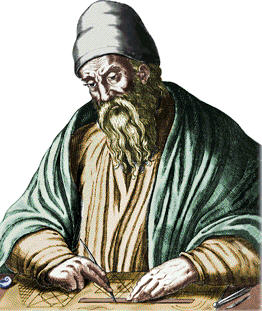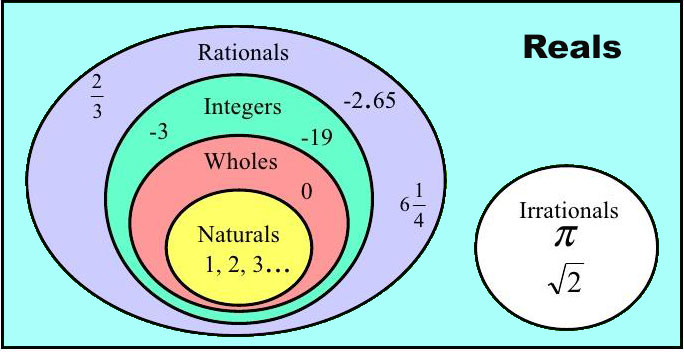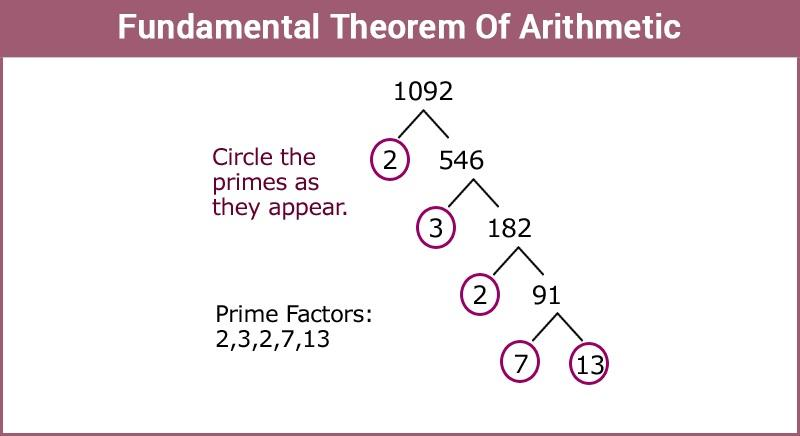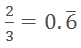×#### Thank you for registering.

One of our academic counsellors will contact you within 1 working day.

Click to Chat

1800-1023-196

+91-120-4616500

CART 0

• 0

MY CART (5)

Use Coupon: CART20 and get 20% off on all online Study Material

ITEM
DETAILS
MRP
DISCOUNT
FINAL PRICE
Total Price: Rs.

There are no items in this cart.
Continue Shopping• Complete JEE Main/Advanced Course and Test Series
• OFFERED PRICE: Rs. 15,900
• View Details

```Revision Notes on Real Numbers

Euclid’s Division LemmaIt is basically the restatement of the usual division system. The formal statement for this is-

For each pair of given positive integers a and b, there exist unique whole numbers q and r which satisfies the relation

a = bq + r, 0 ≤ r < b, where q and r can also be Zero.

where ‘a’ is a dividend, ‘b' is divisor, ‘q’ is quotient and ‘r’ is remainder.

∴ Dividend = (Divisor x Quotient) + Remainder

Natural Numbers

Non-negative counting numbers excluding zero are known as natural numbers.

i.e. 5, 6, 7, 8,  ……….

Whole numbers

All non-negative counting numbers including zero are known as whole numbers.

i.e. 0, 1, 2, 3, 4, 5, …………….

Integers

All negative and non-negative numbers including zero altogether known as integers.

i.e. ………. – 3, – 2, – 1, 0, 1, 2, 3, 4, …………..Algorithm

An algorithm gives us some definite steps to solve a particular type of problem in a well-defined manner.

Lemma

A lemma is a statement which is already proved and is used for proving other statements.

Euclid’s Division Algorithm

This concept is based on Euclid’s division lemma. This is the technique to calculate the HCF (Highest common factor) of given two positive integers m and n,

To calculate the HCF of two positive integers’ m and n with m > n, the following steps are followed:

Step 1: Apply Euclid’s division lemma to find q and r where m = nq + r, 0 ≤ r < n.

Step 2: If the remainder i.e. r = 0, then the HCF will be ‘n’ but if r ≠ 0 then we have to apply Euclid’s division lemma to n and r.

Step 3: Continue with this process until we get the remainder as zero. Now the divisor at this stage will be HCF(m, n). Also, HCF (m, n) = HCF (n, r), where HCF (m, n) means HCF of m and n.

The Fundamental Theorem of Arithmetic

We can factorize each composite number as a product of some prime numbers and of course, this prime factorization of a natural number is unique as the order of the prime factors doesn’t matter.

HCF of given numbers is the highest common factor among all which is also known as GCD i.e. greatest common divisor.

LCM of given numbers is their least common multiple.

If we have two positive integers  ‘m’ and ‘n’ then the property of their  HCF and LCM will be:

HCF (m, n) × LCM (m, n) = m × n.Rational Numbers

The number ‘s’  is known as a rational number if we can write it in the form of m/n where  ‘m' and ‘n’ are integers and n ≠ 0, 2/3, 3/5 etc.

Rational numbers can be written in decimal form also which could be either terminating or non-terminating. E.g. 5/2 = 2.5 (terminating) and(non-terminating).

Irrational Numbers

The number ‘s’ is called irrational if it cannot be written in the form of m/n, where m and n are integers and n≠0 or in the simplest form, the numbers which are not rational are called irrational numbers. Example - √2, √3 etc.

If p is a prime number and p divides a2 , then p is one of the prime factors of a2 which divides a, where a is a positive integer.

If p is a positive number and not a perfect square, then √n is definitely an irrational number.

If p is a prime number, then √p is also an irrational number.

Rational Number and their Decimal Expansions

Let y be a real number whose decimal expansion terminates into a rational number which we can express in the form of a/b, where a and b are coprime, and the prime factorization of the denominator b has the powers of 2 or 5 or both like 2n5m, where n, m are non-negative integers.

Let y be a rational number in the form of y = a/b, so that the prime factorization of the denominator b is of the form 2n5m, where n, m are non-negative integers then y has a terminating decimal expansion.

Let y = a/b be a rational number, if the prime factorization of the denominator b is not in the form of 2n2m, where n, m are non-negative integers then y has a non-terminating repeating decimal expansion.

The decimal expansion of every rational number is either terminating or a non-terminating repeating.

The decimal form of irrational numbers is non-terminating and non-repeating.

```### Course Features

• 728 Video Lectures
• Revision Notes
• Previous Year Papers
• Mind Map
• Study Planner
• NCERT Solutions
• Discussion Forum
• Test paper with Video Solution